maths partner

•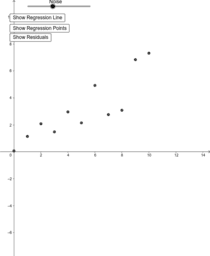Difference between SSE and SSR

Activity

maths partner

•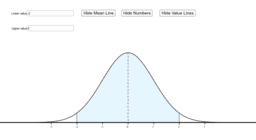Activity

maths partner

•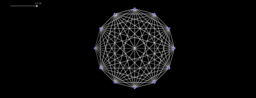kn connected graph

Activity

maths partner

•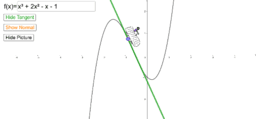Tangents and Normals

Activity

maths partner

•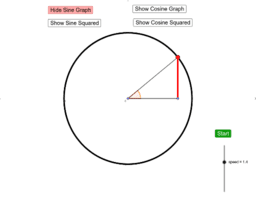Sine and Cosine Squared Graphs on Unit Circle

Activity

maths partner

•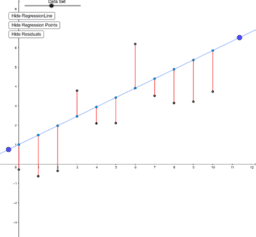Residuals and Regression

Activity

maths partner

•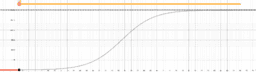Logistic Curve

Activity

maths partner

•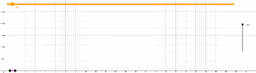COVID19 Virus Data

Activity

maths partner

•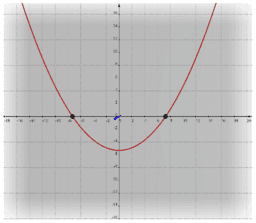Complex Numbers

Book

maths partner

•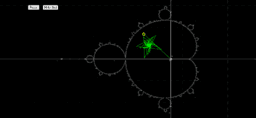Mandelbrot Painter

Activity

maths partner

•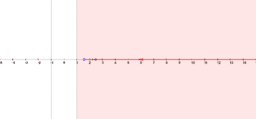Squaring Real Numbers

Activity

maths partner

•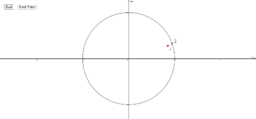Complex Number Raised to Itself

Activity

maths partner

•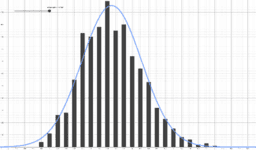Central Limit Theorem

Activity

maths partner

•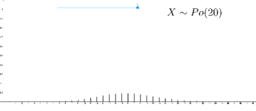Probability Mass Function of a Poisson Distribution

Activity

maths partner

•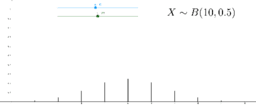Probability Mass Function of a Binomial Distribution

Activity

maths partner

•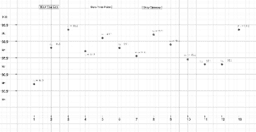Standard Deviation

Activity

maths partner

•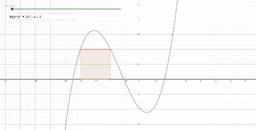Approximating Area Under Curves

Activity

maths partner

•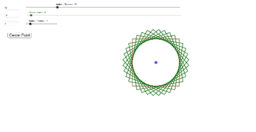Rotate Shapes

Activity

maths partner

•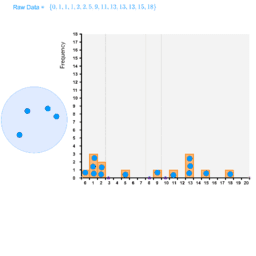Histogram Drag and Drop Points

Activity

maths partner

•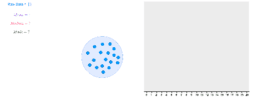Central Tendency with Drag and Drop Data Points

Activity

maths partner

•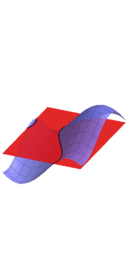Level Curves from 3D Surfaces (different layout)

Activity

maths partner

•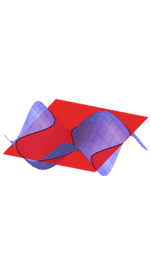Level Curves from 3D Surfaces

Activity

maths partner

•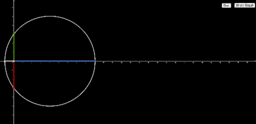Square Root Using Circles

Activity

maths partner

•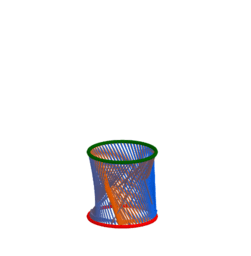2D Functions in 3D

Activity

maths partner